LIFEwork balance We have started a LIFEworkbalance campaign

• Slides: 21LIFE/work balance We have started a #LIFEworkbalance campaign and we need your help to complete our LIFE/work balance survey. We hope to publish the results soon, so please give 15 minutes of your time to help us get a true picture of school life. Want to be a part of this campaign? Take the survey on our website and share it with your colleagues! © Classroom Secrets Limited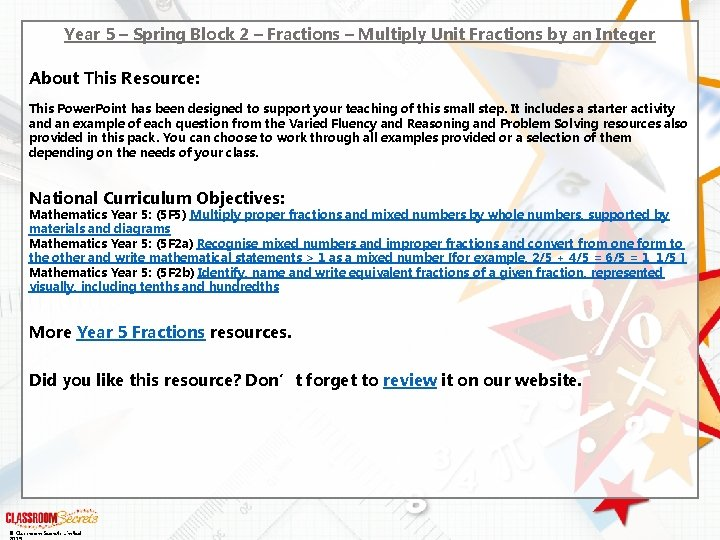Year 5 – Spring Block 2 – Fractions – Multiply Unit Fractions by an Integer About This Resource: This Power. Point has been designed to support your teaching of this small step. It includes a starter activity and an example of each question from the Varied Fluency and Reasoning and Problem Solving resources also provided in this pack. You can choose to work through all examples provided or a selection of them depending on the needs of your class. National Curriculum Objectives: Mathematics Year 5: (5 F 5) Multiply proper fractions and mixed numbers by whole numbers, supported by materials and diagrams Mathematics Year 5: (5 F 2 a) Recognise mixed numbers and improper fractions and convert from one form to the other and write mathematical statements > 1 as a mixed number [for example, 2/5 + 4/5 = 6/5 = 1 1/5 ] Mathematics Year 5: (5 F 2 b) Identify, name and write equivalent fractions of a given fraction, represented visually, including tenths and hundredths More Year 5 Fractions resources. Did you like this resource? Don’t forget to review it on our website. © Classroom Secrets Limited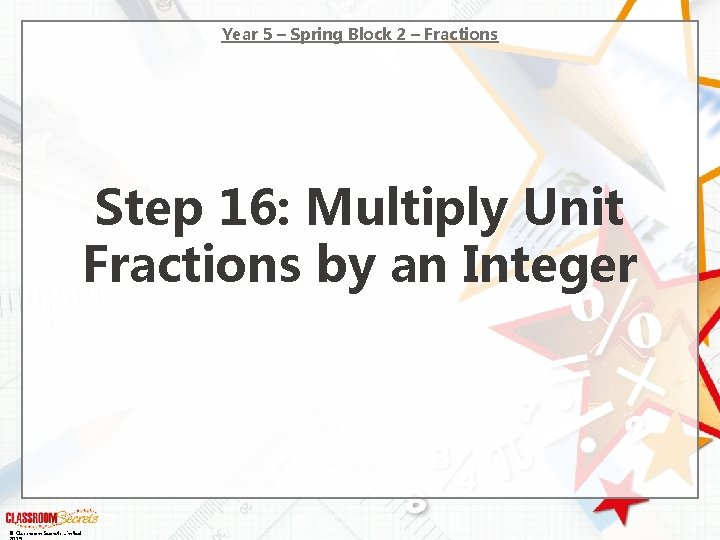Year 5 – Spring Block 2 – Fractions Step 16: Multiply Unit Fractions by an Integer © Classroom Secrets Limited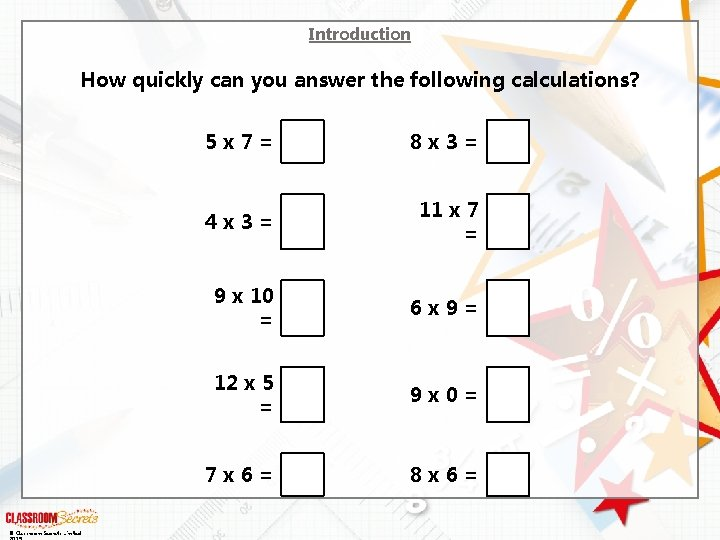Introduction How quickly can you answer the following calculations? © Classroom Secrets Limited 5 x 7 = 8 x 3 = 4 x 3 = 11 x 7 = 9 x 10 = 6 x 9 = 12 x 5 = 9 x 0 = 7 x 6 = 8 x 6 =Introduction How quickly can you answer the following calculations? 5 x 7 = 35 8 x 3 = 24 4 x 3 = 12 11 x 7 77 = 9 x 10 90 = 6 x 9 = 54 12 x 5 60 = 9 x 0 = 7 x 6 = 42 © Classroom Secrets Limited 0 8 x 6 = 48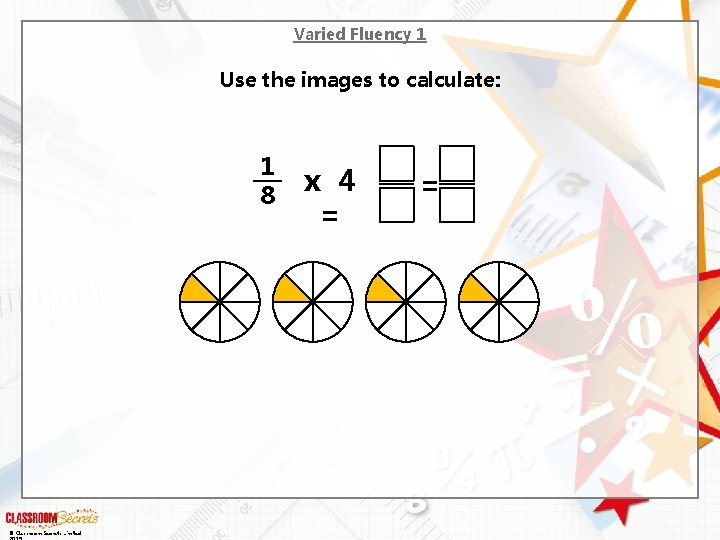Varied Fluency 1 Use the images to calculate: 1 8 © Classroom Secrets Limited x 4 = =Varied Fluency 1 Use the images to calculate: 1 8 © Classroom Secrets Limited 4 1 x 4 = 8 2 =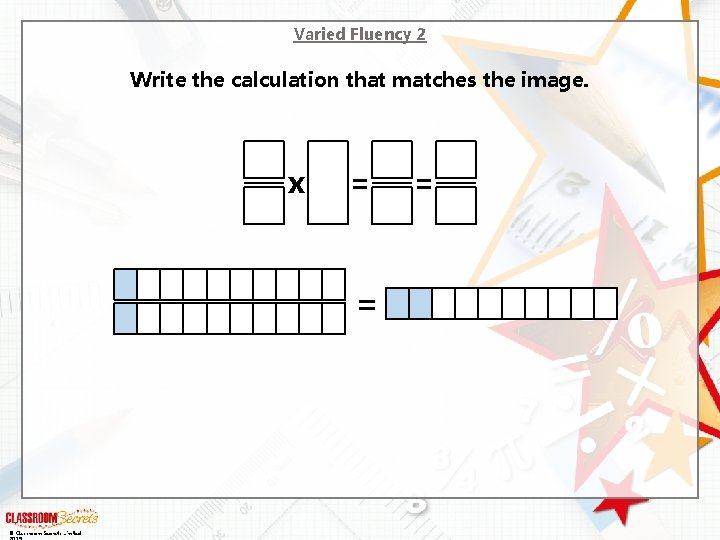Varied Fluency 2 Write the calculation that matches the image. x = = © Classroom Secrets Limited =Varied Fluency 2 Write the calculation that matches the image. 1 10 x 2= 2 10 = © Classroom Secrets Limited = 1 5Varied Fluency 3 Match the correct answer to the calculation below. 1 6 x 4 = A. © Classroom Secrets Limited 1 6 6 4 1 6 4 B. 2 4 1 6 C. 1 6 2 3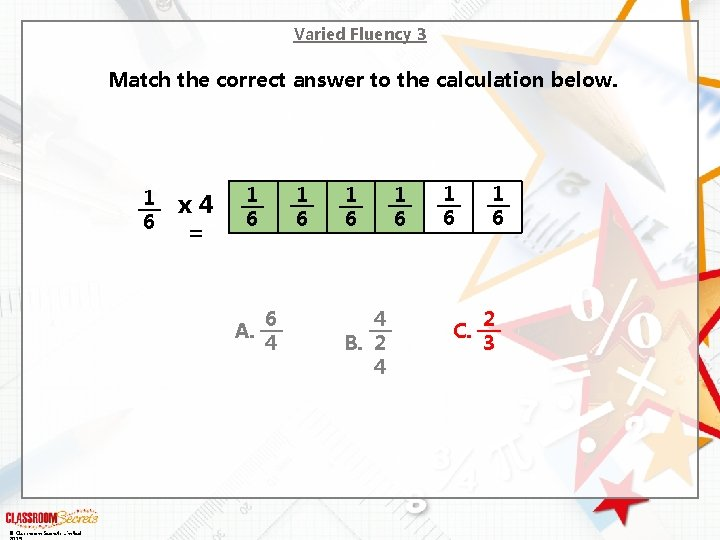Varied Fluency 3 Match the correct answer to the calculation below. 1 6 x 4 = A. © Classroom Secrets Limited 1 6 6 4 1 6 4 B. 2 4 1 6 C. 1 6 2 3Varied Fluency 4 Complete the calculations. © Classroom Secrets Limited 1 7 x = = 1 5 x = =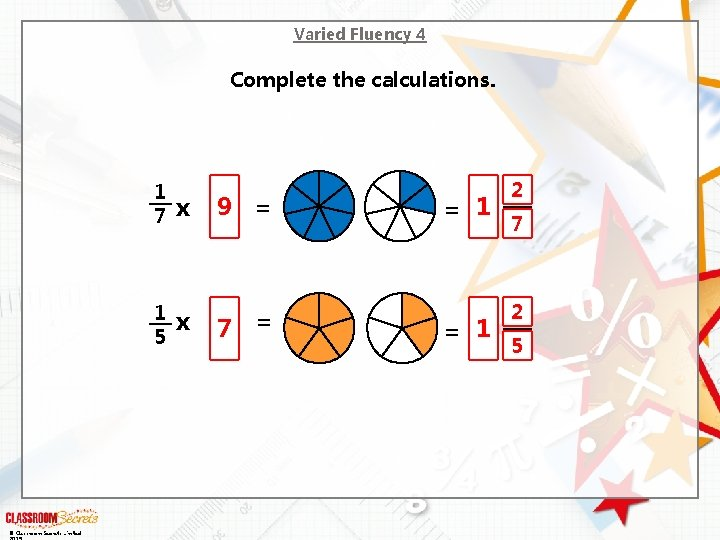Varied Fluency 4 Complete the calculations. © Classroom Secrets Limited 1 7 9 x = 1 5 x = 7 = 1 2 7 2 5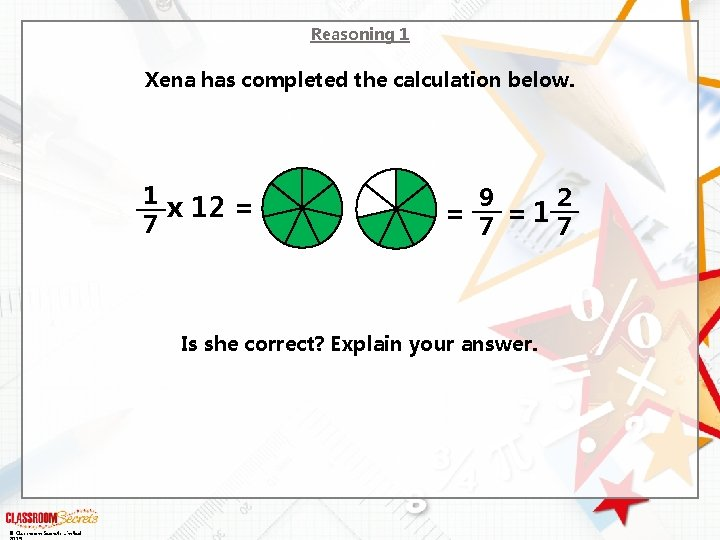Reasoning 1 Xena has completed the calculation below. 1 x 12 = 7 9 2 = 7 =17 Is she correct? Explain your answer. © Classroom Secrets Limited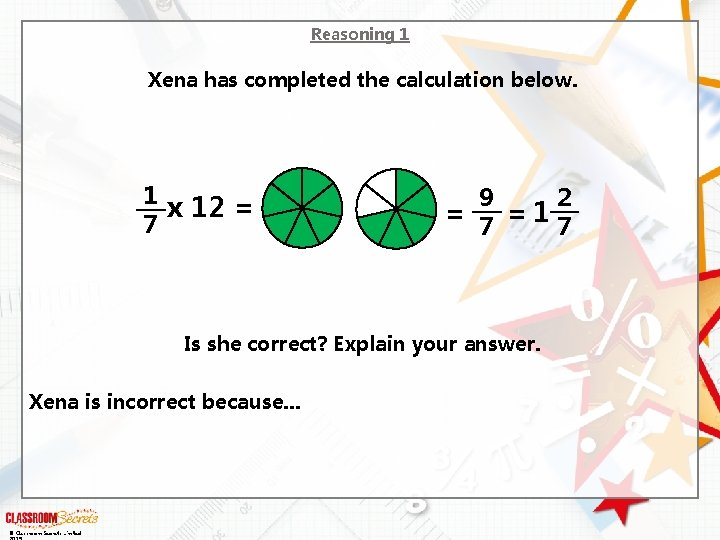Reasoning 1 Xena has completed the calculation below. 1 x 12 = 7 9 2 = 7 =17 Is she correct? Explain your answer. Xena is incorrect because. . . © Classroom Secrets Limited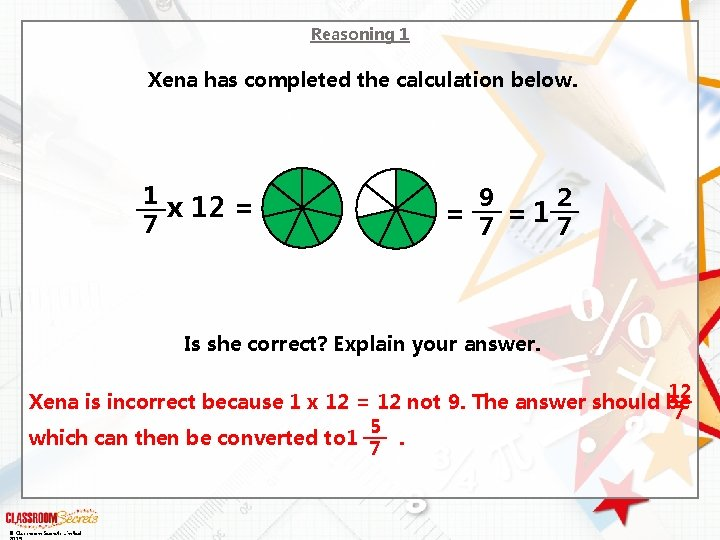Reasoning 1 Xena has completed the calculation below. 1 x 12 = 7 9 2 = 7 =17 Is she correct? Explain your answer. 12 7 Xena is incorrect because 1 x 12 = 12 not 9. The answer should be 5 1 which can then be converted to . 7 © Classroom Secrets Limited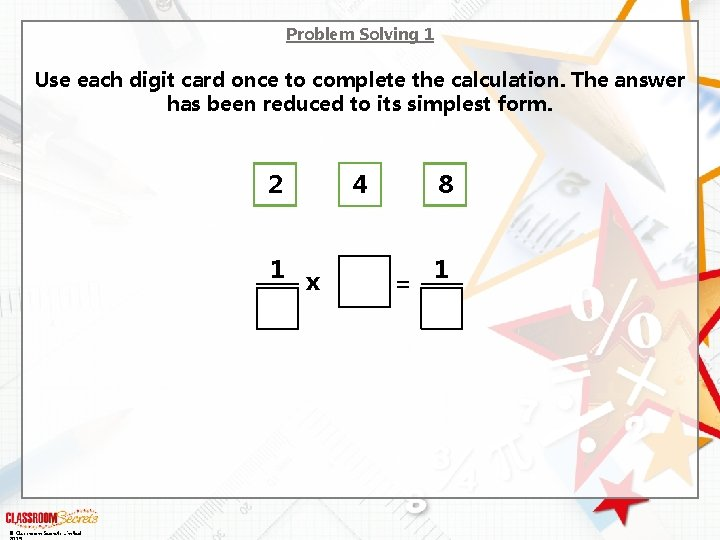Problem Solving 1 Use each digit card once to complete the calculation. The answer has been reduced to its simplest form. 2 1 x © Classroom Secrets Limited 4 8 = 1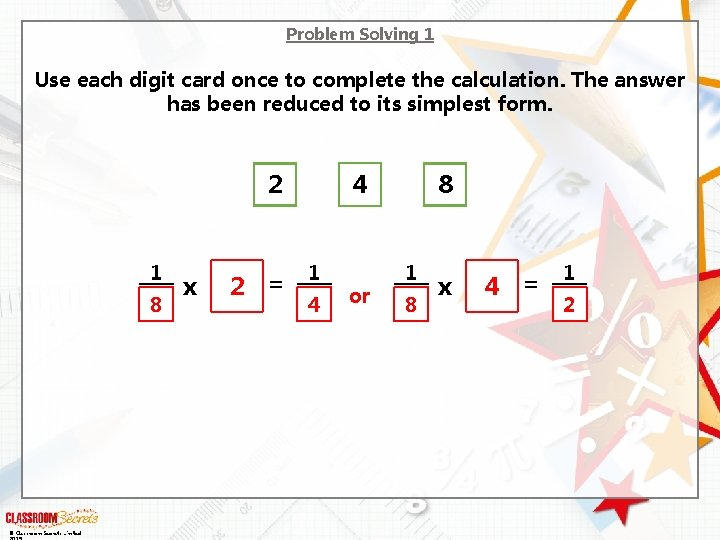Problem Solving 1 Use each digit card once to complete the calculation. The answer has been reduced to its simplest form. 2 1 8 © Classroom Secrets Limited x 2 = 4 1 4 or 8 1 8 x 4 = 1 2Reasoning 2 Solve the problem. 1 Ollie sprints of a mile six times a week. 9 1 Abby sprints of a mile two times a week. 3 Ollie thinks that he sprints further than Abby. Is he correct? Prove it. © Classroom Secrets Limited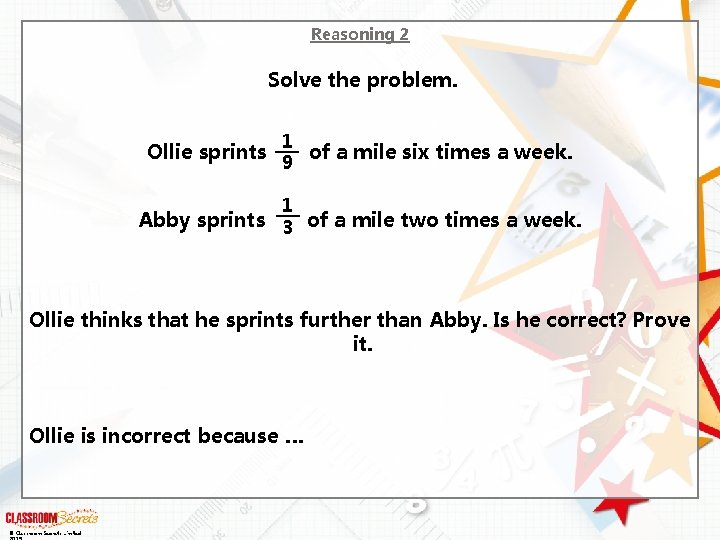Reasoning 2 Solve the problem. 1 Ollie sprints of a mile six times a week. 9 1 Abby sprints of a mile two times a week. 3 Ollie thinks that he sprints further than Abby. Is he correct? Prove it. Ollie is incorrect because … © Classroom Secrets LimitedReasoning 2 Solve the problem. 1 Ollie sprints of a mile six times a week. 9 1 Abby sprints of a mile two times a week. 3 Ollie thinks that he sprints further than Abby. Is he correct? Prove it. 6 9 2 3 Ollie is incorrect because of a mile is the same as of a mile 2 3 as both fractions are equivalent to . © Classroom Secrets Limited